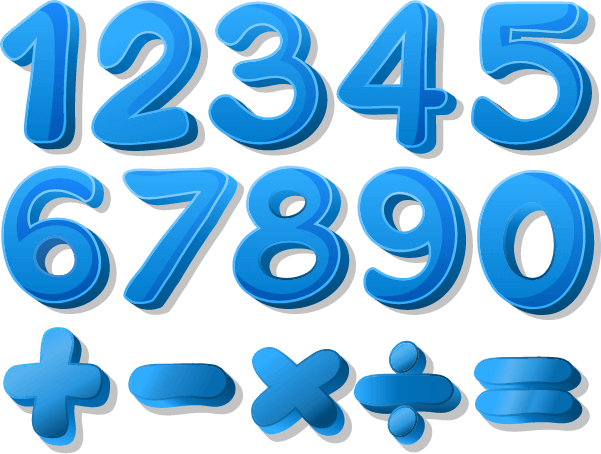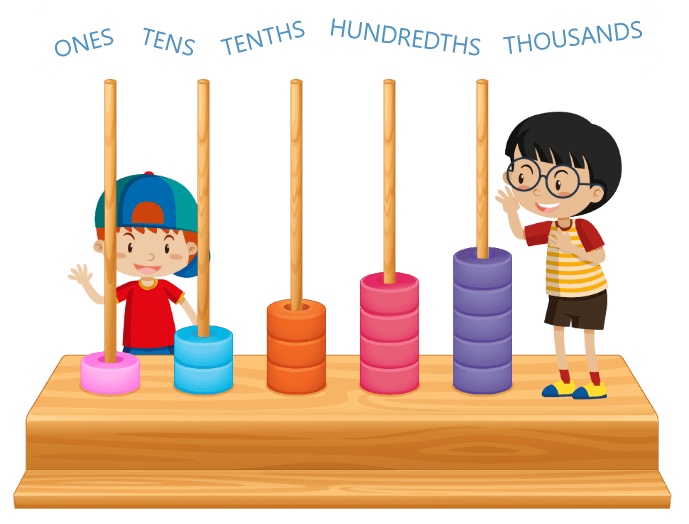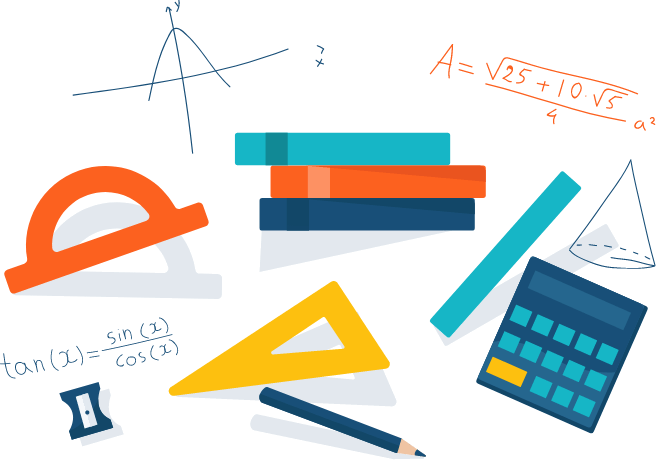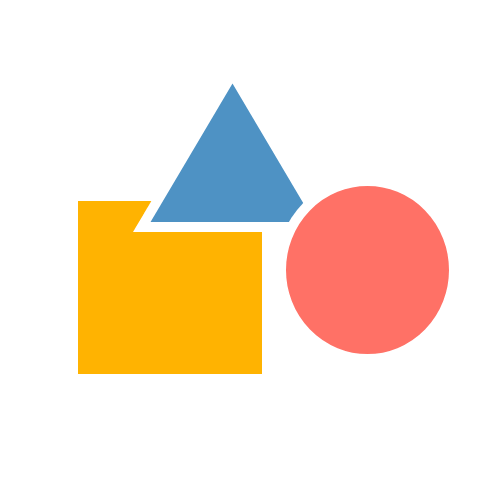# Math Grade - 2 Curriculum

Enter your email for the curriculum
A grade 2 child, at 98th percentile, is exposed to four domains in mathematics as suggested by the Common Core State Standards Initiative. The following is a snapshot of the skills which a child learns in grade 2.
Operations And Algebraic Thinking
Students develop strategies for adding andsubtracting whole numbers after learning numbers. They use a variety of models, including discrete objects and length-based models (e.g., cubes connected to for lengths), to model add-to, take-from, put-together, take-apart, comparesituations to understand the operations of addition and subtraction, and to develop strategies to solve arithmetic problems using these operations. Students understand connections among counting and, addition and subtraction (e.g., adding two is same as counting on two). By comparing a variety of solution strategies, children build their understanding of the relationship between addition and subtraction.Suggested Learning Goals For This Domain

After this domain, student will be able to:

• Represent and solve problems involving addition and subtraction.
• Understand and apply properties of operations and the relationship between addition and subtraction.
• Identify odd and even numbers.
• Add the total number of objects in a rectangular array.Numbers And Operations In Base Ten

Students discuss and develop, strategies to add within 100 and subtract multiples of 10. Students will develop an understanding of problem-solving for numbers up to 100. Students will compose numbers in terms of tens and ones. Students will build number sense to understand orders of counting numbers.

Suggested Learning Goals For This Domain

After this domain, student will be able to:

• Extend the counting sequence.
• Use place value and properties of operations to add and subtract.
• Do calculations mentally using various skills.
Measurement And Data
Students engage in developing an understanding and the meaning of processes of measurement. Students focus both on the transitivity principle for indirect measurement and comparing objects of the equal-sized unit.Suggested Learning Goals For This Domain

After this domain, student will be able to:

• Measure lengths indirectly and by iterating length units.
• Use the relation between different units of measurement
• Tell and write time, with both analog and digital clocks
• Represent and interpret data with help of bar-graphGeometry
Students engage in learning composing and a decomposing plane or solid figures to build an understanding of composite shapes. A student also explores the part-whole relationships between shapes. This also lays a foundation for children's understanding of symmetry and congruence.
Suggested Learning Goals For This Domain

After this domain, student will be able to:

• Reason with shapes and their attributes.
• Calculate the area of rectangular shapess
Features of Math Grade 2 Program

At 98th Percentile, instructional time in Grade 2, focuses on developing an understanding of basic mathematical operations like addition and subtraction, place value, and mathematical logic and reasoning. During this exciting journey, a child is supported through

• A Placement Test aimed at identifying grade-level proficiency in math
• 43 math tutoring sessions
• 5 Benchmark reviews conducted every month
• 4 Revision Classes covering entire syllabus
• An Exit Placement Test
• Final Benchmark Assessment to validate students’ grade-level proficiency

During the 52 tutoring sessions, a teacher ensures that student is prepared to meet the highest standards in math problem solving by supporting the child on daily basis through the following:

• Discussion on Critical Thinking Question
• Individual Skill Gap Analysis and Gap Filling
• Mandatory In-Class practice of concepts learned
• Minimum 30 minutes of daily practice through Homework
• Monthly celebrations based on the event theme merged with Math concepts

During this journey of students, at 98thPercentile, teachers ensure a regular interaction with parents. Parents stay connected through:

• Regular Parent-Teacher meeting aimed at sharing students’ progress
• A repository of recorded videos to help the child at home
• Printable lesson-wise worksheets to provide additional practice to the child
Disclaimer: The number of classes may increase or decrease depending on the discretion of the teacher. If a teacher reads the child has acquired grade specific skills she can decide to accelerate the child resulting in reduction in the number of classes, similarly when a child needs more support to acquire grade specific skills number of classes will go up.